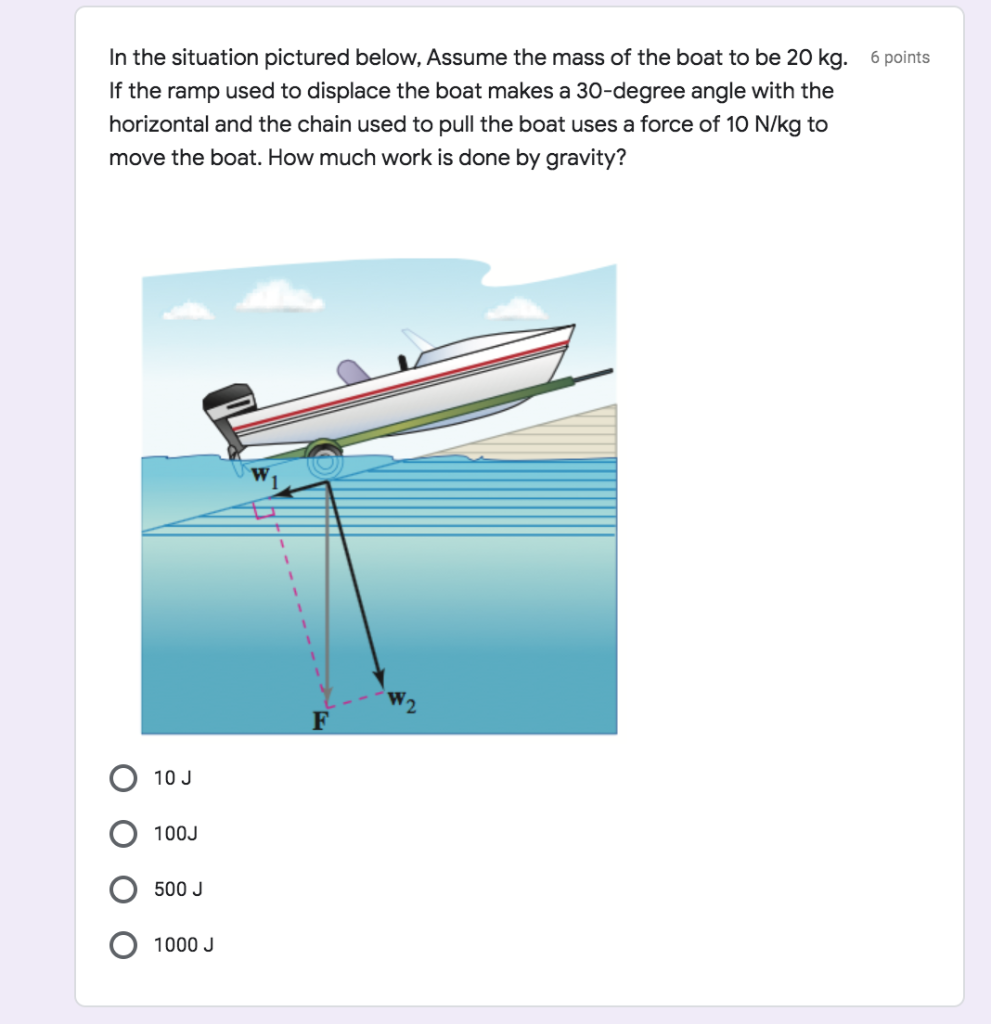# In the situation pictured below, Assume the mass of the boat to be 20 kg. 6...

###### Question:In the situation pictured below, Assume the mass of the boat to be 20 kg. 6 points If the ramp used to displace the boat makes a 30-degree angle with the horizontal and the chain used to pull the boat uses a force of 10 N/kg to move the boat. How much work is done by gravity? F 10 J 100J 500 J 1000 J